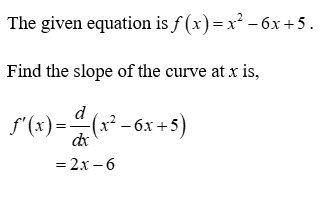# Finding Slope locationslet f(x)=x^2-6x+5a. Find the Values of x for which the slope of the curve y=f(x) is 0b. Find the values of x for which the slope of the curve y=f(x) is 2

Question
2 views

Finding Slope locations

let f(x)=x^2-6x+5

a. Find the Values of x for which the slope of the curve y=f(x) is 0

b. Find the values of x for which the slope of the curve y=f(x) is 2

check_circle

Step 1

Consider the given equation....

### Want to see the full answer?

See Solution

#### Want to see this answer and more?

Solutions are written by subject experts who are available 24/7. Questions are typically answered within 1 hour.*

See Solution
*Response times may vary by subject and question.
Tagged in